ISEE Lower Level Quantitative : Numbers and Operations

Example Questions

← Previous 1 3 4 5 6 7 8 9 208 209

Example Question #1 : Isee Lower Level (Grades 5 6) Quantitative Reasoning

Sandy made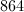candles in one day.  If she packs them in boxes of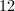, how many boxes will she have?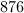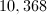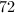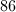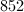Explanation:

Sandy hascandles, which she needs to divide in to boxes that containcandles each.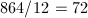Sandy has 72 boxes of candles.

Example Question #2 : Isee Lower Level (Grades 5 6) Quantitative Reasoning

Find the quotient.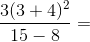21

10

147

18

21

Explanation:

When solving a problem with multiple operations, always remember: PEMDAS!

To find the quotient, first do the "P"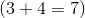.

Then the "E"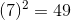Then the "M"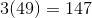Now "D"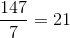The quotient is 21.

Example Question #1 : Isee Lower Level (Grades 5 6) Quantitative Reasoning

Which expression is equivalent to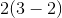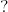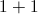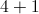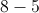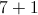Explanation:

The original expression is equal to 2 (remember order of operations-PEMDAS). Therefore,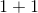is the correct answer because that is the only response that is equal to 2.

Example Question #4 : Isee Lower Level (Grades 5 6) Quantitative Reasoning

In the pantry, there is an equal number of cereal boxes, bags of chips, and boxes of cookies. How many items could be in the pantry?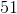Explanation:

In order to have an equal number of cereal, chips, and cookies, the number of total items needs to be a number that is divisble by three. Of the four answer choices,is the only number that is a multiple of three.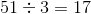Therefore,is the correct answer choice.

Example Question #1 : Isee Lower Level (Grades 5 6) Quantitative Reasoning

Alex typeswords per minute. How many words does she type per second?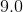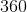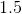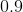Explanation:

Since there are sixty seconds in a minute, divide the number of words Alex types per minute by sixty to see how many she types per second.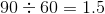Alex typeswords per second.

Example Question #6 : Isee Lower Level (Grades 5 6) Quantitative Reasoning

Ifcan be divided by bothand, it can also be divided by which number without leaving a remainder?Explanation:

Of the four answer choices given,is the only choice that has bothandas factors. Therefore, any number that is a multiple of, for example,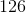or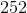, is also divisible by bothand.

Example Question #2 : Isee Lower Level (Grades 5 6) Quantitative Reasoning

Maxine has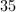graham crackes that she would like to share equally amoung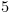friends.  Which expression shows how many graham crackers each friend will recieve?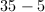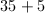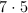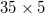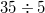Explanation:

Since Maxine has 35 graham crackers that she is going to split equaly amoung 5 friends, she must divide.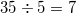Example Question #8 : Isee Lower Level (Grades 5 6) Quantitative Reasoning

One British pound is worth approximately $1.61. For how many British pounds (nearest whole) can a tourist expect to exchange$1,000?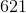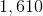The correct answer is not among the other choices.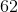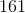Explanation:

\$1,000 is equivalent to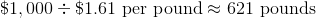Example Question #1 : Isee Lower Level (Grades 5 6) Quantitative Reasoning

Which of the following is NOT true about the number 18?

It is divisble by 3.

It is divisible by 12.

It is divisible by 18.

It is divisble by 6.

It is divisible by 12.

Explanation:

While the number 18 is divisible by 3, 6, and 18, it is not divisible by 12.

Dividing 18 by 12 results in a remainder of 6.

Therefore, the statement that is not true about the number 18 (and is hence the correct answer choice) is "It is divisible by 12."

Example Question #2 : Isee Lower Level (Grades 5 6) Quantitative Reasoning

Which of the following is a factor of 24?